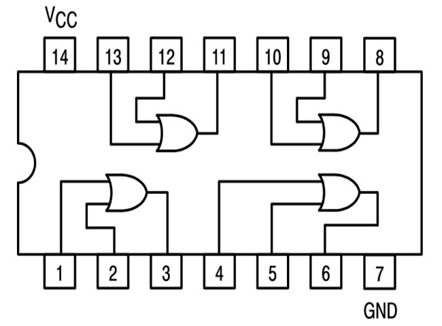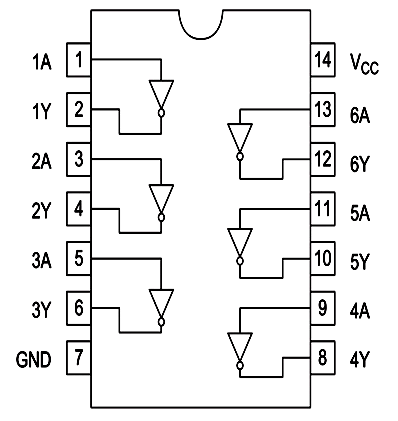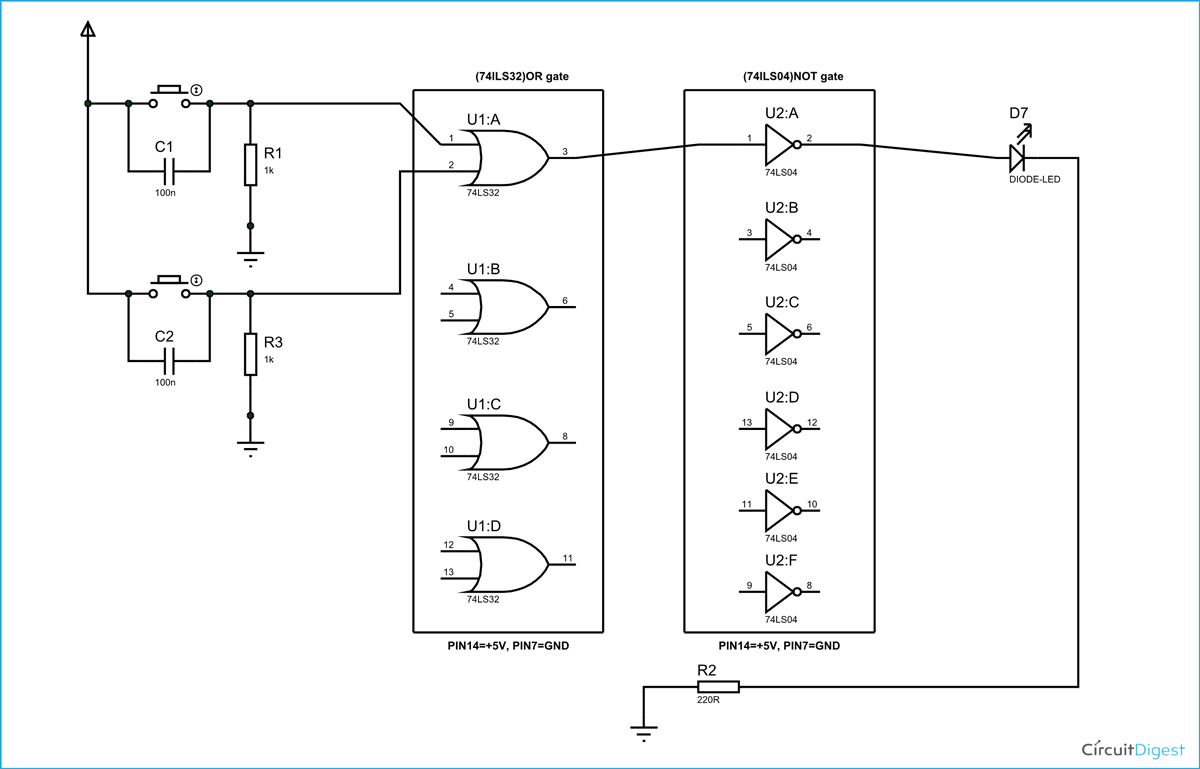NOR gate is a digital logic gate, designed for arithmetic and logical operations. This gate is mainly used in applications where there is a need for mathematical calculations. So in calculators, computers and many digital applications use this gate. Here we are going to use 74LS32 IC for demonstration, this chip has 4 OR gates in it. These four gate are connected internally as shown in below figure.These gates have limitations for working voltage and input logic frequency. When these limitations are not considered the chip may damage permanently, so one should pay attention while selecting the  logic gates. These OR gates are followed by NOT gates. NOR gates provide inverted output of OR gate, so with both, we will have NOR gate. Here we use 74LS04 HEX NOT gate IC.### Components

Power supply (5v), 1K resistor (2 pieces), 220Ω resistor, 74LS32 QUAD ORGATE IC, 74LS04 HEX NOT gate IC, 1 LEDS, buttons (2 pieces), 100nF capacitor (2 pieces) Connecting wires, breadboard.

### Circuit Diagram and Working Explanation

The truth table of NOR gate is shown in below figure:

 A B Output 0 0 1 0 1 0 1 0 0 1 1 0

As in truth table the output of a NOR gate should be HIGH only if both the gate inputs are LOW. In any other case the output should be LOW. So if any one or both inputs are HIGH,the output of NOR gate will be LOW.

In this nor gate circuit we are going to pull down both input of a gate to ground through a 1KΩ resistor.  And then the inputs are connected to power through a button.

So when the button is pressed the corresponding pin of gate INPUT goes high. So with two buttons we can realize the truth table of NOR gate. When one of button is pressed one input of gate will be high and other will be low at this time the output should be LOW. When both buttons are released, both inputs will be LOW, at this time the OUTPUT of OR gate will be HIGH.

When both buttons are pressed, both INPUTS of OR will be HIGH, at this time OUTPUT should be LOW. So by truth table, the LED should be ON only when both buttons are released. If any or either buttons are released the LED should be OFF.These pull down resistors are necessary as the chosen Integrated Circuit is a positive edge triggering one. If the resistors are ignored the circuit might generate unpredictable results.

The capacitors here are for neutralizing the bouncing effect of buttons. Although the capacitors here are not compulsory, putting them might smooth the working of gate.

Video

Subscribe below to receive most popular news, articles and DIY projects from Circuit Digest

•noor
Jan 29, 2016

thanks

•tushar sharma
Oct 31, 2016

nor gate

•GOURAV
Feb 16, 2018

THANKS FOR DETAILS OF NOR GATE